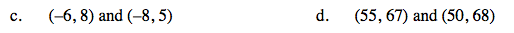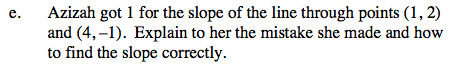### Home > AC > Chapter 7 > Lesson 7.2.3 > Problem7-75

7-75.
1.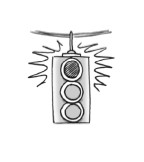Find the slope of the line passing through each pair of points below. Homework Help ✎

1. (1, 2) and (4, −1)

2. (7, 3) and (5, 4)

3. (−6, 8) and (−8, 5)

4. (55, 67) and (50, 68)

5. Azizah got 1 for the slope of the line through points (1, 2) and (4, −1). Explain to her the mistake she made and how to find the slope correctly.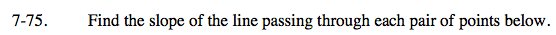Either graph the two points to make a slope triangle or subtract the x-values from each other and the y-values from each other and substitute them into the slope formula.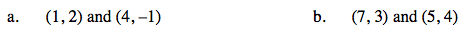Δx: 1 − 4 = −3
Δy: 2 − (−1) = 3

$\text{slope }=\frac{\Delta y}{\Delta x}=\frac {3}{-3}$

slope = −1

$-\frac{1}{2}$

Use the same strategy to solve parts (b) through (e).